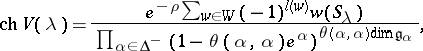# Difference between revisions of "Weyl-Kac character formula"

Weyl–Kac formula, Kac–Weyl character formula, Kac–Weyl formula, Weyl–Kac–Borcherds character formula

A formula describing the character of an irreducible highest weight module (with dominant integral highest weight) of a Kac–Moody algebra. The formula is a generalization of Weyl's classical formula for the character of an irreducible finite-dimensional representation of a semi-simple Lie algebra (cf. Character formula). The formula is very robust and has been steadily applied (with increasing technical complications) to the representations of ever wider classes of algebras, see [a3] for representations of Kac–Moody algebras and [a2] for generalized Kac–Moody (or Borcherds) algebras.

Let $\frak g$ be a Borcherds (colour) superalgebra (cf. also Borcherds Lie algebra) with charge $\underline{m}$ and integral Borcherds–Cartan matrix $A = ( a _ { ij} )$, restricted with respect to the colouring matrix $C$. (The charge counts the multiplicities of the simple roots.) Let $\mathfrak h$ denote the Cartan subalgebra of $\frak g$ and let $V$ be a weight $\frak g$-module with all weight spaces finite-dimensional. The formal character of $V$ is

\begin{equation*} \operatorname { ch } V = \sum _ { \mu \in \mathfrak{h} ^ { * } } ( \operatorname { dim } V _ { \mu } ) e ^ { \mu }. \end{equation*}

For $V ( \lambda )$ an irreducible highest weight module with dominant integral highest weight $\lambda$, U. Ray [a6] and M. Miyamoto [a5] have established the following generalization of the Weyl–Kac–Borcherds character formula.

Let $W$ be the Weyl group, $\Delta^{-}$ the negative roots and $R$ the set of simple roots counted with multiplicities. Let $\rho \in \mathfrak { h } ^ { * }$ be such that

\begin{equation*} \rho ( h _ { i } ) = \frac { 1 } { 2 } a _ { i i } \end{equation*}

for all $i$. Define $S _ { \lambda } = e ^ { \lambda + \rho } \sum _ { \gamma } ( - 1 ) ^ { | \gamma | } e ^ { - \gamma }$, where the sum runs over all elements of the weight lattice of the form $\gamma = \sum _ { i = 1 } ^ { r } \alpha _ { i } + \sum _ { j = 1 } ^ { s } p _ { j } \beta _ { j }$ such that the $\alpha _ { k }$ are distinct even imaginary roots in $R$, the $\beta_l$ are distinct odd imaginary roots in $R$,

\begin{equation*} ( \alpha _ { k } | \alpha _ { l } ) = ( \beta _ { k } | \beta _ { l } ) = 0 \end{equation*}

if $k \neq l$,

\begin{equation*} ( \alpha _ { k } | \beta _ { l } ) = 0 \end{equation*}

for all $k$, $l$,

\begin{equation*} ( \beta _ { k } \mid \beta _ { k } ) = 0 \end{equation*}

if $p _ { k } > 1$, and

\begin{equation*} ( \lambda | \alpha _ { k } ) = ( \lambda | \beta _ { l } ) = 0 \end{equation*}

for all $k$, $l$. Set $r = s = 0$ if $\beta = 0$, and define $| \gamma | = r + \sum _ { j = 1 } ^ { s } p _ { j }$. Thenwhere $\theta$ is the colouring map induced by $C$ and $\mathfrak { g } _ { \alpha }$ is the $\alpha$ root space of $\frak g$.

In the case of Kac–Moody algebras, there are no imaginary simple roots and $\theta ( \alpha , \alpha ) = 1$ for all $\alpha$, so one recovers the Weyl–Kac formula

\begin{equation*} \operatorname { ch } V ( \lambda ) = \frac { \sum _ { w \in W } ( - 1 ) ^ { l ( w ) } e ^ { w ( \lambda + \rho ) - \rho } } { \prod _ { \alpha \in \Delta ^ { - }} ( 1 - e ^ { \alpha } ) ^ { \text{dim} \mathfrak{g} _ { \alpha } } }. \end{equation*}

These character formulas may also be applied to representations of associated quantum groups where quantum deformation theorems are known (see [a4] and [a1], for example).

How to Cite This Entry:
Weyl-Kac character formula. Encyclopedia of Mathematics. URL: http://encyclopediaofmath.org/index.php?title=Weyl-Kac_character_formula&oldid=23135
This article was adapted from an original article by Duncan J. Melville (originator), which appeared in Encyclopedia of Mathematics - ISBN 1402006098. See original article